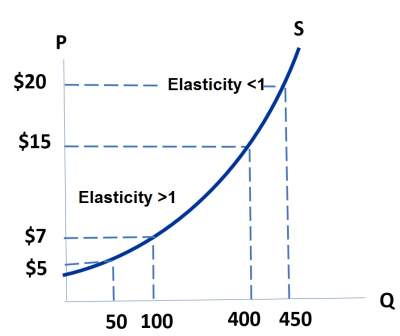# Price Elasticity of Supply

## Introduction

Price Elasticity of Supply = (% change in Qs) / (% change in P)
• Price elasticity of supply determines how much Qs responds to a change in P.
• Basically, it determines sellers' price-sensitivity.
• The midpoint method is used to compute the % changes.

### Example

Let's assume that price elasticity of supply = 24% / 8% = 3% (see figure below).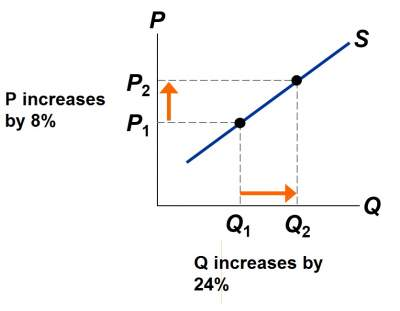### The Variety of Supply Curves

• The slope of the supply curve is closely related to price elasticity of supply.
• Usually, the flatter the curve, the bigger the elasticity. The steeper the curve, the smaller the elasticity.
• 5 different classifications will be discussed

### Perfectly Inelastic Supply

Price Elasticity of Supply = (% change in Qs) / (% change in P) = 0% / 15% = 0
 S curve: vertical Sellers' price sensitivity: none Elasticity: 0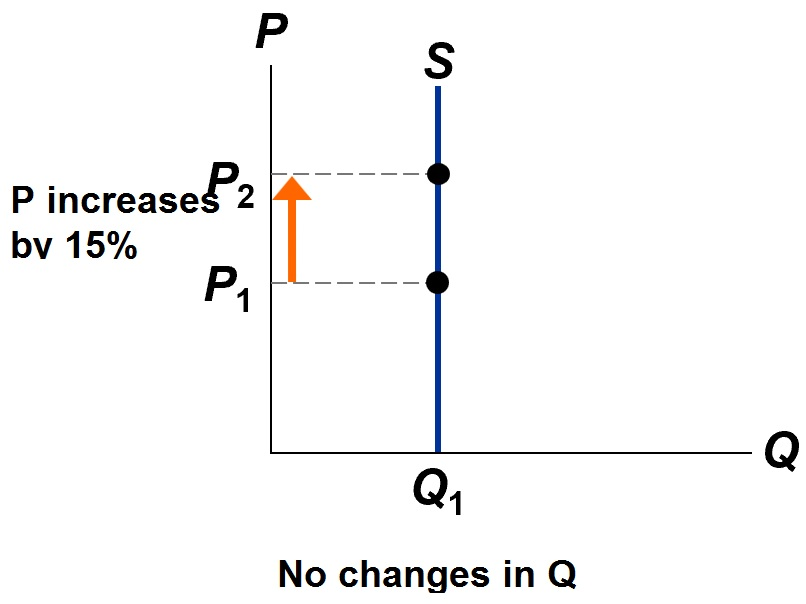### Inelastic Supply

Price Elasticity of Supply = (% change in Qs) / (% change in P) = < 15% / 15% < 1
 S curve: relatively steep Sellers' price sensitivity: relatively low Elasticity: < 1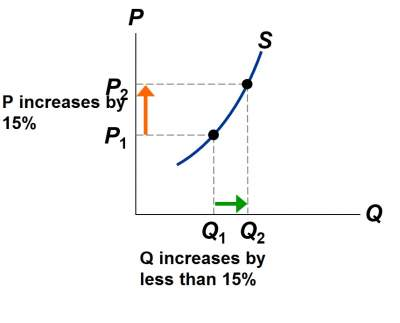### Unit Elastic Supply

Price Elasticity of Supply = (% change in Qs) / (% change in P) = 15% / 15% = 1
 S curve: intermediate slope Sellers' price sensitivity: intermediate Elasticity: = 1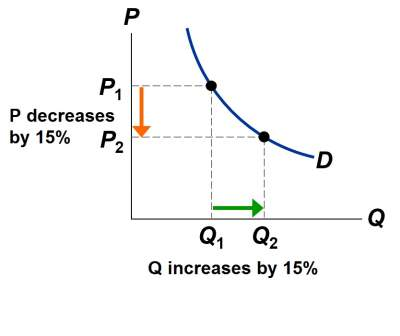### Elastic Supply

Price Elasticity of Supply = (% change in Qs) / (% change in P) = (> 15%) / (15%) > 1
 S curve: relatively flat Sellers' price sensitivity: relatively high Elasticity: > 1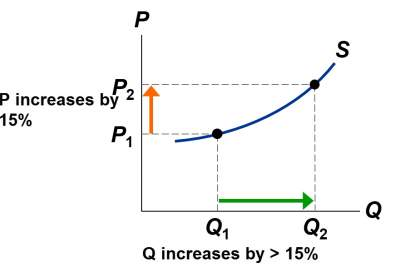#### Perfectly Elastic Supply

Price Elasticity of Supply = (% change in Qs) / (% change in P) = (any value) / (0%) = ∞
 S curve: horizontal Sellers' price sensitivity: extreme Elasticity: ∞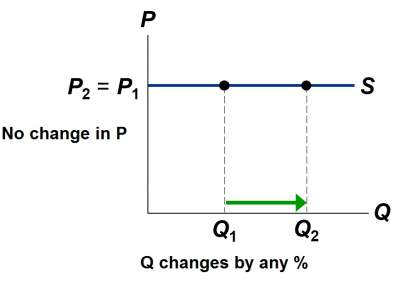### The Determinants of Supply Elasticity

• The easier sellers can change the quantity they produce, the greater the price elasticity of supply.
• Example: Supply of oil or electricity is harder to vary and therefore less elastic than supply of cars.

### Exercises

Exercise on Elasticity and Changes in Equilibrium

Check your answers here: Solution to the Exercise on Elasticity and Changes in Equilibrium

### How the Price Elasticity of Supply Can Vary

Supply usualy eventually be less elastic when Q increases, because of its capacity limits (see figure below).